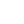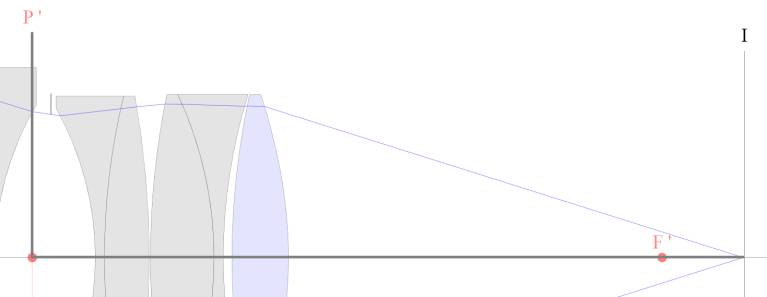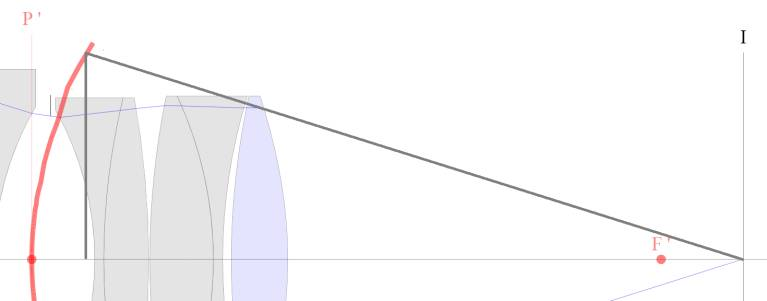----------- Your trusted source for independent sensor data- Photons to Photos------------ Last revised: 2020-09-11 19:00 GMT-5

# ---------------------------- Optics Primer - Sine versus Tangent

--------------------------------------------------------- By Bill Claff

Astute readers may have noticed that sometimes we use sine in formulas, such as for Numeric Aperture (NA), and other times we use tangent, such as for angle of view.

We can examine the geometry to get a better understanding of this.

For example, we might think that an angle from the optical axis on the image plane back toward something like the exit pupil would look like this:I've added dark gray lines to indicate the sides that would form the tangent.

But, the pupils, and principal "planes" are in fact curved, particularly for a well corrected lens.
A classic example is the Abbe sine condition; the details of which I omit here.
In this case the situation looks more like this:Now the dark gray lines indicate the sides that would form the sine. This is the case for Numeric Aperture (NA).

The example I've chosen is imperfect because no lens in perfectly corrected but this illustrates the concept.

To summarize; angles starting on the optical axis and running to a plane use tangent whereas those running to a curved surface (assumed spherical) use sine.